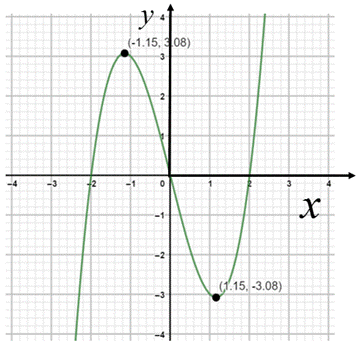# To graph the function using graphing device.### Precalculus: Mathematics for Calcu...

6th Edition
Stewart + 5 others
Publisher: Cengage Learning
ISBN: 9780840068071### Precalculus: Mathematics for Calcu...

6th Edition
Stewart + 5 others
Publisher: Cengage Learning
ISBN: 9780840068071

#### Solutions

Chapter 2.3, Problem 24E

a.

To determine

## To graph the function using graphing device.

Expert Solution

### Explanation of Solution

Given information : Use graphing device to graph the function f(x)=x34x .

Graph : Sketch the graph using graphing utility.

Step 1: Press WINDOW button to access the Window editor.

Step 2: Press Y= button.

Step 3: Enter the expression x34x which is required to graph.

Step 4: Press GRAPH button to graph the function.

The graph is obtained as:Interpretation :

From the above graph, it can be observed that the domain of f is and the range is .

b.

To determine

### To state approximately the interval on which the function is increasing and on which the function is decreasing.

Expert Solution

The function f is decreasing on (,2.5] and increasing on [2.5,) .

### Explanation of Solution

Given information :

The function is f(x)=x34x .

The graph of the function is obtained as:Using TRACE feature on the calculator, the lowest value is f(1.15)=3.08 and f(1.15)=3.08 .

From the above graph, it can be observed that the function f is decreasing on [1.15,1.15] and increasing on (,1.15] and [1.15,] .

### Have a homework question?

Subscribe to bartleby learn! Ask subject matter experts 30 homework questions each month. Plus, you’ll have access to millions of step-by-step textbook answers!